International
Tables for
Crystallography
Volume H
Powder diffraction
Edited by C. J. Gilmore, J. A. Kaduk and H. Schenk

International Tables for Crystallography (2018). Vol. H, ch. 2.4, pp. 103-104

## Section 2.4.2. Electron powder diffraction pattern geometry and intensity

J.-M. Zuoa and J. L. Lábára

### 2.4.2. Electron powder diffraction pattern geometry and intensity

| top | pdf |

The powder diffraction rings in transmission geometry appear where the cone of diffracted electron beams intersects the Ewald sphere. The intersection creates a ring of diffracted beams, which is then projected onto the planar detector (see Fig. 2.4.2) with a radius (R) according to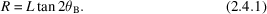Here θB is the Bragg diffraction angle and L is the camera length.Figure 2.4.2 | top | pdf |Schematic diagram of the Ewald sphere construction and the geometry for recording electron diffraction patterns.

The d-spacing can be obtained by measuring the length of R in an experimental diffraction pattern using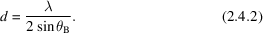The electron wavelength is determined by the electron accelerating voltage (Φ), in volts: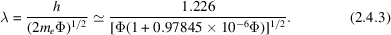The wavelength of high-energy electrons is relatively short. For 200 kV electrons, the wavelength is 0.025 Å and the Bragg angle is very small. For example, for d = 2.5 Å the electron scattering angle θ is 5 mrad. For a small Bragg angle one can use the approximation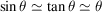. This gives the relationship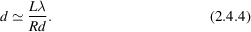At large scattering angles with≃ 2 Å−1 or greater, a better approximation is given by (Cowley & Hewat, 2004)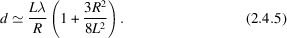The camera length L can be determined using a sample with known d-spacings, while the electron wavelength or acceleration voltage can be calibrated using high-order Laue zone (HOLZ) lines in convergent-beam electron diffraction (CBED) patterns (Zuo, 1993).

For a small parallelepiped crystal fully illuminated by a coherent electron beam of intensity, the kinematic diffraction intensity is given by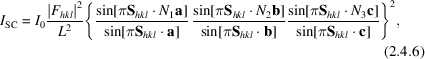where N1, N2 and N3 are the number of unit cells along the three axis directions, and Fhkl is the electron structure factor of the hkl reflection: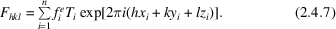Here T is the atomic displacement factor, which accounts for atomic thermal vibrations, and the electron atomic scattering factor fie is defined by equation (4.3.1.13)in International Tables for Crystallography, Vol. C (2004). For a reflection with the scattering vectorthe deviation from the Bragg condition of the hkl reflection is expressed by the excitation error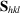: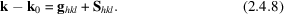The diffraction intensity recorded in a powder diffraction pattern is the integrated intensity over the crystal orientation and the detector area. A change in crystal orientation leads to a change in the excitation error normal to the diffracted beam in the plane of Bragg reflection. The integration in these three directions is equivalent to integration over the reciprocal-space volume around the Bragg peak. The result gives the diffraction power of a sample with a large number of crystallites for the hkl reflection as (Warren, 1990)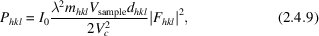where Vsample is the sample volume, mhkl is the multiplicity of the reflection based on the symmetry-equivalent number of hkl reflections, andis the volume of the unit cell. For randomly oriented powder samples, the diffraction power is uniformly distributed over the bottom edge of a cone of half apex angle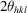and height L, and the peak intensity is more appropriately described by the power per unit length of the diffraction circle (Vainshtein, 1964):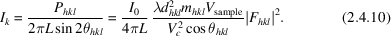Here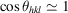is a good approximation for electron diffraction and this formula is presented in equation (2.4.1.3)in International Tables for Crystallography, Vol. C (2004).

The kinematic approximation in electron diffraction is valid only for very small crystals. Defining the validity of the kinematic approximation for different crystals has been difficult and the subject of extensive debate (Blackman, 1939; Vainshtein, 1964; Turner & Cowley, 1969; Cowley, 1995). For single-crystal electron diffraction, numerous studies using CBED have demonstrated an almost perfect fit to experimental diffraction intensities using dynamic theory. Using this fitting approach, experimental structure-factor amplitudes and phases can be measured through a refinement process with high accuracy (Saunders et al., 1995; Tsuda et al., 2002; Zuo, 2004). However, this approach requires knowledge of the approximate crystal structure and can rarely be used for powder electron diffraction, where unknown crystal structures are often studied. In developing a theory for the integrated intensity for powder electron diffraction, the magnitude of the dynamic effect and its dependence on crystal orientations, defects, thickness variations and crystal shape must be considered. In X-ray and neutron diffraction, the combination of these factors led to the highly successful kinematical theory of ideal imperfect crystals with randomly distributed mosaic blocks. For electron diffraction, an all-encompassing theory of integrated intensity has been elusive because of the small electron coherence length, which is much less than the size of typical mosaic blocks detected by X-ray and neutron diffraction, and strong scattering. An approximation has been developed to take account of dynamical scattering using the two-beam theory (Blackman, 1939). Under this approximation, the integrated dynamic intensity Id over a large range of excitation is given by the expression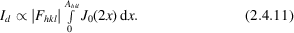Here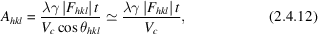where t is the thickness of the crystallite along the electron-beam direction, γ is the relativistic constant of electrons and J0(2x) is the zero-order Bessel function. For a very small value of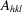the Bessel function J0(2x) is nearly constant with a value of 1 and the diffraction intensity approaches that of the kinematical limit. From this, the following formula can be derived for the dynamical intensity: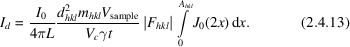For very large Ahkl, the integral over the Bessel function approaches the value of 1/2 and in this case the diffraction intensity is proportional to the structure-factor amplitude instead of its square as predicted by kinematical theory.

The extent of dynamic effects that can be reduced by averaging over crystal orientations has been demonstrated by precession electron diffraction (PED). This technique was originally developed by Vincent & Midgley (1994) to improve the single-crystal electron diffraction intensities for structural analysis. In PED, the incident electron beam is tilted and precessed along a conical surface that is centred on the electron optical axis. Below the crystal, the diffraction pattern is tilted back with the position of the direct beam remaining approximately constant during precession. The diffraction pattern then generally appears similar to a conventional electron diffraction pattern. The measured diffraction intensity, however, is a double integration over the two-dimensional detector and the incident-beam angles defined by the precession cone surface. Experimental and theoretical studies of PED integrated intensities have shown an overall `more kinematical' behaviour with less sensitivity to crystal thickness and exact orientation than for conventional electron diffraction patterns. Simulations also showed that the dynamical effects are still present in the PED integrated intensities, but the extent of the dynamic effect as measured by the correlation between the integrated intensity and the squared amplitude of the structure factor follows the empirical rules:

 (i) The correlation increases with the precession angle. (ii) The correlation is more pronounced for higher-order reflections than lower-order ones, for which the integration over the different excitation error is less complete. (iii) The correlation also improves as the crystal thickness decreases.

In the electron powder diffraction of randomly oriented crystals, the angular integration is performed over the entire solid angle. Zone-axis patterns with enhanced dynamical interaction between the diffracted beams are also included in this solid angle. However, the overall probability for a crystal to be in exact zone-axis orientation is very small, even if the zone axis is defined within a wedge of tens of milliradians. Thus, powder electron data generally tend to be more kinematical than single-crystal data.

### References

Blackman, M. (1939). On the intensities of electron diffraction rings. Proc. R. Soc. London Ser. A, 173, 68–82.Google Scholar
Cowley, J. M. (1995). Diffraction Physics. Amsterdam: Elsevier Science BV.Google Scholar
Cowley, J. M. & Hewat, A. W. (2004). Powder and related techniques: electron and neutron techniques. International Tables for Crystallography Vol. C, pp. 80–83. Dordrecht: Kluwer Academic Publishers.Google Scholar
International Tables for Crystallography (2004). Volume C, Mathematical, Physical and Chemical Tables, edited by E. Prince. Dordrecht: Kluwer Academic Publishers.Google Scholar
Saunders, M., Bird, D. M., Zaluzec, N. J., Burgess, W. G., Preston, A. R. & Humphreys, C. J. (1995). Measurement of low-order structure factors for silicon from zone-axis CBED patterns. Ultramicroscopy, 60, 311–323.Google Scholar
Tsuda, K., Ogata, Y., Takagi, K., Hashimoto, T. & Tanaka, M. (2002). Refinement of crystal structural parameters and charge density using convergent-beam electron diffraction – the rhombohedral phase of LaCrO3. Acta Cryst. A58, 514–525.Google Scholar
Turner, P. S. & Cowley, J. M. (1969). The effects of N-beam dynamical diffraction on electron diffraction intensities from polycrystalline materials. Acta Cryst. A25, 475–481.Google Scholar
Vainshtein, B. K. (1964). Structure Analysis by Electron Diffraction. Oxford: Pergamon Press.Google Scholar
Vincent, R. & Midgley, P. A. (1994). Double conical beam-rocking system for measurement of integrated electron diffraction intensities. Ultramicroscopy, 53, 271–282.Google Scholar
Warren, B. E. (1990). X-ray Diffraction. New York: Dover Publications.Google Scholar
Zuo, J. M. (1993). New method of Bravais lattice determination. Ultramicroscopy, 52, 459–464.Google Scholar
Zuo, J. M. (2004). Measurements of electron densities in solids: a real-space view of electronic structure and bonding in inorganic crystals. Rep. Prog. Phys. 67, 2053–2103.Google Scholar